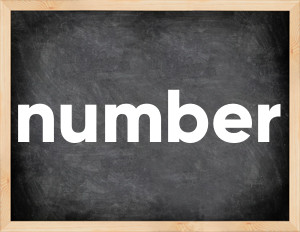# Number past tenseThe English verb 'number' is pronounced as [ˈnʌmbər].
Related to: regular verbs.
3 forms of verb number: Infinitive (number), Past Simple - (numbered), Past Participle - (number).

## Here are the past tense forms of the verb number

👉 Forms of verb number in future and past simple and past participle.
❓ What is the past tense of number.

## Number: Past, Present, and Participle Forms

Base Form Past Simple Past Participle
number [ˈnʌmbər]

numbered [ˈnʌmbəd]

number [ˈnʌmbəd]

## What are the 2nd and 3rd forms of the verb number?

🎓 What are the past simple, future simple, present perfect, past perfect, and future perfect forms of the base form (infinitive) 'number'?

### Learn the three forms of the English verb 'number'

• the first form (V1) is 'number' used in present simple and future simple tenses.
• the second form (V2) is 'numbered' used in past simple tense.
• the third form (V3) is 'number' used in present perfect and past perfect tenses.

## What are the past tense and past participle of number?

The past tense and past participle of number are: number in past simple is numbered, and past participle is number.

### What is the past tense of number?

The past tense of the verb "number" is "numbered", and the past participle is "number".

### Verb Tenses

Past simple — number in past simple numbered (V2).
Future simple — number in future simple is number (will + V1).
Present Perfect — number in present perfect tense is number (have/has + V3).
Past Perfect — number in past perfect tense is number (had + V3).

### number regular or irregular verb?

👉 Is 'number' a regular or irregular verb? The verb 'number' is regular verb.

## Examples of Verb number in Sentences

•   It's so stupid for a woman to number that among her talents (Present Simple)
•   Our days are numbered (Present Simple)
•   Our crew and passengers have been numbered 845 (Present Perfect)
•   I asked students to number the questions from 1 to 10 (Past Simple)
•   Their party numbered thirty in all (Past Simple)
•   The population numbers two thousand (Present Simple)
•   She was numbered with the saints (Past Simple)
•   He numbered me among his friends (Past Simple)
•   This book is numbered among the treasures of the library (Present Simple)
•   I didn't manage to number the houses (Past Simple)

Along with number, words are popular get out and conquer.

Verbs by letter: , , , , , , , , , , , , , , , , , , , , , , , , .